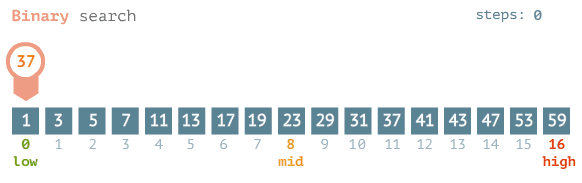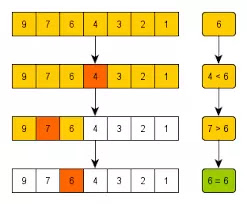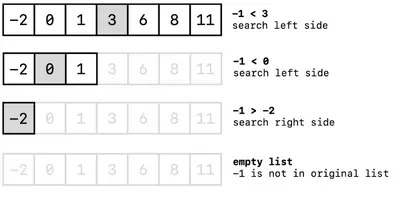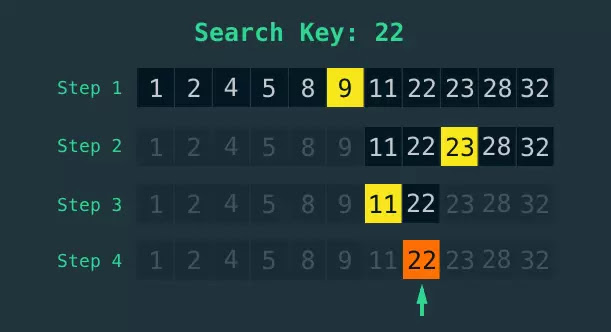What is Binary search

Binary search is another fairly intuitive way of searching for things. It's a very efficient algorithm.It's a logarithmic algorithm and it's intuitive if you imagine you were searching through an actual physical dictionary.

Binary Search relies upon the list that you're searching to be sorted.Just like a dictionary is sorted.

How Binary search works

Real life example

So imagine you had a dictionary in front of you and you wanted to search for the word "ostrich". You wouldn't start at page one of the dictionary and then go to page 2 and then page 3 and then page 4.You'd turn to sort of somewhere in the middle and then you'd be able to discard all of the letters to the left all of the pages to the left as you turn to them to the middle and then you'd be able to get another section sort of somewhere halfway between the stack that you have your breath you cut that in half and you'd get that page and then if the O's were on that side you'd keep hold of the bit in your left hand or if they were you'd keep hold of the bit in your right hand and you'd keep repeating that process until you found the word that you were looking for.

Binary search algorithm

a binary search does something very similar. So it relies on having a sorted list so you have this list. Let's say it's 1024 items long. And what you do with binary search is you go to the middle item of this sorted list and if that's the item you're looking for then that's it.The search is over.

if the item is not the item you're looking for but is higher than the item that you're looking for in value in numerical value or in the latter value depending what it is that you're searching for. Then you can say well the item that I'm looking for must be in the left part of the list because I know these are in the correct order they're sorted.So if I've landed on the midpoint of this list and I'm the item I land on is higher in value than the item I'm looking for.I can get rid of all of the items to the right of me to the to the right of the item that I've landed on because I know that they are also going to be too high. And then you repeat that process.

But in doing that first step you get rid of half of the items that you're searching through so your list of 1024 items is now 512 items and then you repeat that process.But the bit of the list that you've got left you go to the midpoint of your sorted list and you determine whether that's the item you're looking for. If it's not you ask whether it's higher or lower than the item you're looking for.

If it's higher than you get rid of all the items that are to the right all the items that are higher than the item you're on. And if it's lower you get rid of all of the items in the other direction. so you've just halved your search again You've halved the number of items that you have to search through again.

So now we're down to 256 and then you keep repeating this process the next time you do it you're down to 128 items and then you're down to 64 items and then 32 items and then 16 and then 8 then for them too until eventually you you reach the item that you're looking for . it's the only item that's left.

You keep repeating that process until you find the item you're looking for. And by doing so each time you're cutting the number of items that you'd have to search through by half. So each time the length of the list is halving and then halving and then halving and then halving again until it gets down to just one item which is either the item you're looking for or it's not the item  it doesn't exist.Binary search in Python

And so you can see it's a very efficient searching algorithm but it does rely on the list being sorted. So anyway we're going to code a binary search in Python now binary search algorithm so you can see exactly  how it works right. Binary search then. So we have our holding function here.

def binary_search(item,my_list):
pass

So we have a function called binary search it also like linear search takes the item that you're looking for and the name of the list .We need to know whether we found the item we're looking for.

So to begin with we haven't found it. we'll set it to False but that will change if we find what we're looking for. And we also need to keep track of the first item in the list. And the last item in the list. Mainly so that we can calculate the midpoint of the list because we are going to move to the midpoint of the list as we go through and carry out our binary search.

So if we create a variable and call it first and set that to zero and then we create a variable and we call that last and we set that variable to the last item in the list. Now that's going to be the length of the list which is my list minus 1 because of the zero indexing that we have in Python.

def binary_search(item,my_list):
found=False
first=0
last=len(my_list)-1

And then we're going  to create a loop that will keep us searching until we've either found what we're looking for or we've exhausted all the search options and we haven't returned anything at all. We haven't found the item we're looking for.

And so what would the condition be for that. Well we want to search while certain conditions are met. So it would be a while loop that we're using and the conditions that we need to ensure are true that  :

• we need the first item to be less than or equal to the last item. So that is as our list gets shorter because we keep dividing it in two. We need to make sure that the first and last are in the right order because they're eventually going to converge to one value. And so that's why we need that.
• we need found to be false because we don't want to continue our search. If we found what we're looking for.

So those are the conditions and then we now need to calculate what our midpoint will be because we're going to be moving to our midpoint. And so the midpoint is just equal to the first place And the last place divided by two but we'll do floor division because we don't want a decimal point because we can't have a decimal point for an index. So if we do floor division there that will give us our midpoint and it will give us our midpoint every time as this list gets shorter and shorter as we iterate through the search.

def binary_search(item,my_list):
found=False
first=0
last=len(my_list)-1
while first <=last and found==False:
midpoint=(first+last)//2

Now the first thing we need to check is if the item at the midpoint is the item we're looking for then we found our item.So we need to make sure that we account for that. So if the midpoint equals the item I'm looking for then job done. I found what I'm looking for and found equals true.

if my_list[midpoint]==item:
found=True

But if it doesn't we need to either look on the left hand side or on the right hand side and the conditions for that are.

if the value at the midpoint is less than the item that we're looking for then the new list starts on the right hand side. It starts at the next point up so we can say that first now equals the midpoint plus 1. And similarly in sort of reverse logic if you like otherwise the last item is equal to the midpoint minus what's minus 1. Because we're then concerned with just the items on the left hand side if midpoint is higher than the item that we're looking for because remember we have a sorted list here.

if my_list[midpoint]==item:
found=True
else:
if my_list[midpoint]<item:
first=midpoint+1
else:
last=midpoint-1

So we know that to be true. And that said we just keep iterating through that until we find or don't find the item we're looking for. And when we get to the end of the search we return found and that will let us know whether or not we found the item we're looking for.

def binary_search(item,my_list):
found=False
first=0
last=len(my_list)-1
while first <=last and found==False:
midpoint=(first+last)//2
if my_list[midpoint]==item:
found=True
else:
if my_list[midpoint]<item:
first=midpoint+1
else:
last=midpoint-1
return foundTesting our python binary search function

We will test the code on three lists

list1=[1,2,4,5,8,9,11,22,23,28,32]
list2=[99,298,300,587]
list3=[-9,-2,6,33]
print(binary_search(22,list1))
print(binary_search(7,list1))
print(binary_search(300,list2))
print(binary_search(-2,list3))

Output

True
False
True
True

Conclusion

That's how you implement binary search in Python. And I would definitely urge you to practice that and keep keep thinking about it and keep coding it and keep writing that code until you've really got it fixed in your mind how it works and then you can almost write the code with your eyes closed and follow the logic each time. And that will really help that understanding of how binary search works and it'll just become second nature to you.